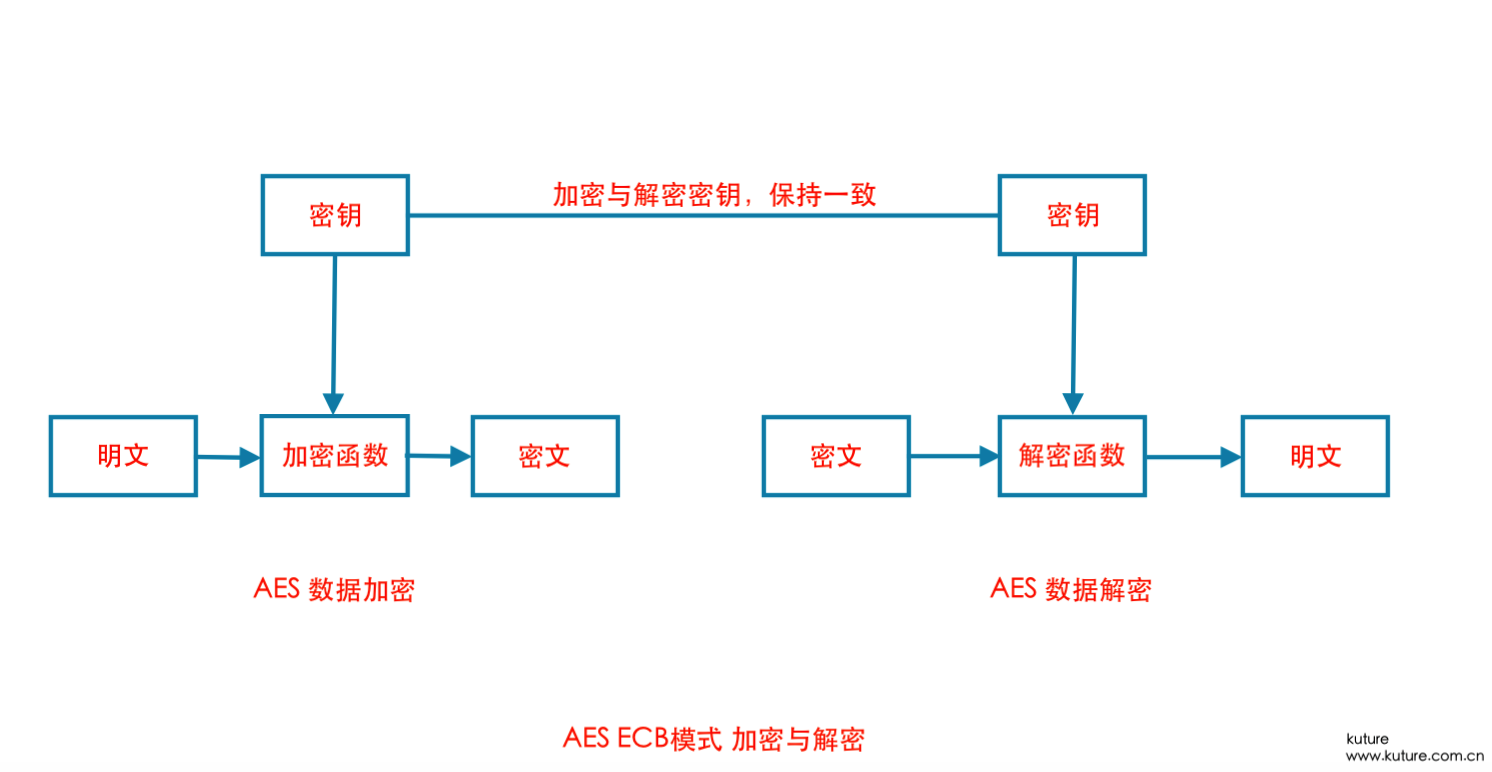2020/08/14 - 次.

# 基于AES ECB算法的文件加密与解密### 加密原理

AES加密过程是在一个4×4的字节矩阵上运作，这个矩阵又称为“状态（state）”，其初值就是一个明文区块（矩阵中一个元素大小就是明文区块中的一个Byte）。（Rijndael加密法因支持更大的区块，其矩阵行数可视情况增加）加密时，各轮AES加密循环（除最后一轮外）均包含4个步骤：

• SubBytes — 通过非线性的替换函数，用查找表的方式把每个字节替换成对应的字节。
• ShiftRows —将矩阵中的每个横列进行循环式移位。

### 代码实现

from Crypto.Cipher import AES
from binascii import b2a_hex, a2b_hex

# model file aes encrypt and decrypt
class AESModelEncryptDecrypt(object):

# aes key complement 16 with zero

if isinstance(text, str): text = text.encode()
if len(text) % 16:add = 16 - (len(text) % 16)
text = text + ('\13'.encode() * add)
return text

# aes encrypt
def aes_encrypt(self, en_text, model_aes_key):

mode = AES.MODE_ECB
cryptos = AES.new(key, mode)
cipher_text = cryptos.encrypt(text)
return b2a_hex(cipher_text)

# aes decrypt
def aes_decrypt(self, de_text, model_aes_key):
mode = AES.MODE_ECB
cryptor = AES.new(key, mode)
plain_text = cryptor.decrypt(a2b_hex(de_text))
return plain_text.rstrip('\13'.encode())

with open(model_name, 'rb') as rf:
rf.close()
return contents, model_name

# model file encrypt
def model_file_encrypt(self, contents, model_name):
model_encrypt = self.aes_encrypt(contents, MODELAESKEY)
with open(model_name, 'wb') as sf:
pickle.dump(model_encrypt, sf)

# mode file decrypt
def model_file_decrypt(self, model_name):
with open(model_name, 'rb') as rf:
rf.close()
model_decrypt = self.aes_decrypt(model_encrypt, MODELAESKEY)
return model_decrypt

# aes model file save
def model_file_save(self, conetents, model_name):

with open(model_name + '.decrypt_temp', 'wb') as sf:
sf.write(conetents)
sf.close()
return model_name + '.decrypt_temp'